# What is Capacitance?

• Last Updated : 21 Jul, 2021

A capacitor is an electrical component with a two-terminal that may store energy in the form of an electric charge. It is made up of two electrically conducting plates separated by a certain distance. The space between the conductors can be filled with a vacuum or a dielectric, which is an insulating substance.

Capacitance refers to the capacitor’s ability to store charges. Capacitors store energy by separating opposing charge pairs. A parallel plate capacitor is the most basic form, consisting of two metal plates separated by a gap. However, capacitors come in a variety of shapes, sizes, lengths, and girths, as well as a variety of materials.

### Capacitor and Capacitance

A capacitor is a two-conductor arrangement with an insulator between them.

Charges Q1 and Q2 as well as potentials V1 and V2 are present in the conductors. In practice, the two conductors usually have charges Q and – Q, with a potential difference between them of V= V1 – V2. Only this type of capacitor charge setup will be considered. (By assuming the other conductor is at infinity, even a single conductor can be utilized as a capacitor.) By connecting the conductors to the two terminals of a battery, the conductors can be charged in this way. Though Q is referred to as the capacitor’s charge, it actually refers to the charge on one of the conductors; the capacitor’s overall charge is zero.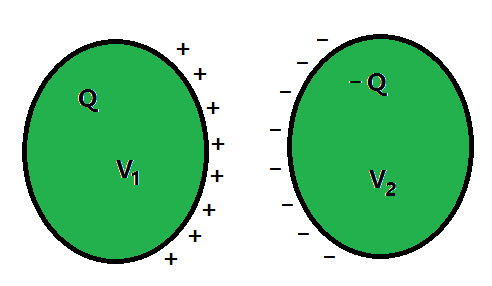The charge Q is proportional to the electric field in the region between the conductors.

That is, if the capacitor’s charge is doubled, the electric field will be doubled at all points. (This is due to Coulomb’s law and the superposition principle, which imply a straight proportionality between field and charge.) The work done per unit positive charge in taking a little test charge from the conductor 2 to 1 against the field is now known as the potential difference V.

Consequently, V is also proportional to Q, and the ratio Q/V is a constant can be written as:

C = Q / V                                                                                                                                                                                                            ………(1)

The constant C is called the capacitance of the capacitor. C is independent of Q or V, as stated above.

The capacitance C depends only on the geometrical configuration of a two-conductor system (shape, size, separation). It also depends on the nature of the insulator (dielectric) that separates the two conductors.

1 farad is the SI unit of capacitance (=1 coulomb volt-1) or 1 F = 1 C V–1. The sign for a capacitor with fixed capacitance (a) and the symbol for one with variable capacitance (b) is drawn in the figure below.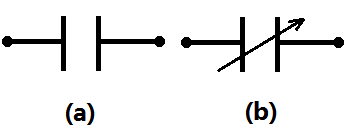Equation (1) demonstrates that for a given Q, V is small for a big C. This means that a capacitor with high capacitance can store a big quantity of charge Q at a low voltage. This is crucial in the real world. The presence of a strong electric field around the conductors is implied by a large potential difference. A high electric field can ionize the surrounding air and speed the charges to the oppositely charged plates, at least partially neutralizing the charge on the capacitor plates. In other words, the capacitor’s charge leaks out due to the intervening medium’s reduced insulating power.

• The dielectric strength of a dielectric medium is the greatest electric field that it can withstand without losing its insulating ability; for air, it is around 3 × 106 Vm–1
• This field corresponds to a potential difference of 3× 104 V between the conductors over a distance of 1 cm or less between them.
• To hold a considerable quantity of charge without leaking, a capacitor’s capacitance must be large enough that the potential difference, and hence the electric field, do not exceed the break-down limits.

Standard Units of Capacitance are:

• To put it another way, there is a limit to how much charge can be stored on a capacitor without considerable leakage. 1 farad is the SI unit of capacitance.
• A farad is a very large unit in practice; the most common sub-multiples are 1 µF = 10–6 F, 1 nF = 10–9 F, 1 pF = 10–12 F, and so on.
• A capacitor is a crucial component of most ac circuits having vital functions in addition to retaining a charge.

### Working of a Capacitor

Consider the simplest basic capacitor structure: the parallel plate capacitor. It is made up of two parallel plates that are separated by a dielectric layer. One plate is attached to the positive end (plate I) and the second plate is connected to the negative end (plate II) when a DC voltage source is placed across the capacitor (plate II). Plate I becomes positive in relation to plate II when the battery’s voltage is applied across the capacitor. The current tries to pass through the capacitor from its positive plate to its negative plate in the steady-state condition. However, due to the separation of them with an insulating layer, it is unable to flow.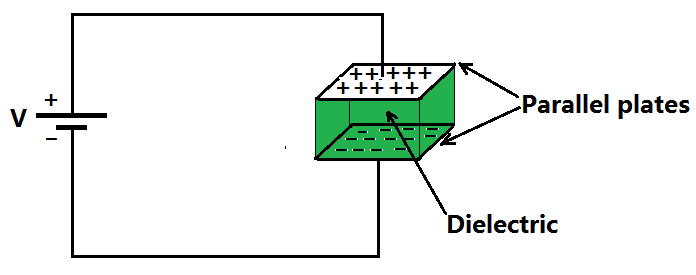In the capacitor, an electric field appears between the parallel plates. The positive charge is collected on the plate connected to the positive terminal of the battery, while the negative charge is collected on the plate connected to the negative terminal of the battery. After a certain point, the capacitor’s capacitance with respect to this voltage allows it to collect the maximum amount of charge. The capacitor’s charging time is defined as the time it takes to charge the capacitor.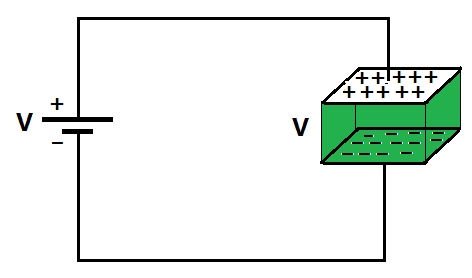The two plates of the capacitor keep a negative and positive charge for a period of time after the battery is removed. As a result, the capacitor serves as a power source. If these plates are linked to a load, current flows from Plate I to Plate II to the load until all charges on both plates have been dissipated. The capacitor’s discharge time is the duration of this time span.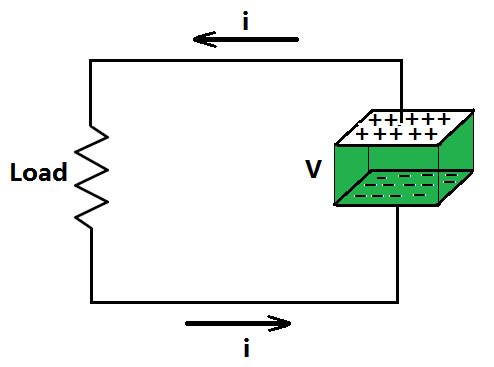### Formula for Capacitance

The conducting plates have charges q1 and q2 (normally, if one plate has a charge of +q, the other plate has a charge of –q). The charge applied to the conducting plates determines the electric field in the region between the plates. The electric field is proportional to the potential difference (V).

Therefore, the expression for the capacitance can be written as

Q ∝ V

Q = CV

C = Q / V

This constant of proportionality is well called the capacitance of the capacitor.

Depending on the use, a capacitor’s capacitance might be constant or variable. It may appear from the equation that ‘C’ is dependent on charge and voltage. Actually, it is dependent on the capacitor’s form and size, as well as the insulator employed between the conducting plates.

### Sample Questions

Problem 1: A spherical capacitor has an inner sphere of radius 12 cm and an outer sphere of radius 13 cm. The outer sphere is earthed and the inner sphere is given a charge of 2.5 µC. The space between the concentric spheres is filled with a liquid of dielectric constant 32. Determine the capacitance of the capacitor.

Solution:

Given:

The radius of the inner sphere,  r2 is 12 cm that is 0.12 m.

The radius of the outer sphere, r1 is 13 cm that is 0.13 m.

Charge on the inner sphere, q is  2.5 μC that is 2.5 x 10-6 C.

Dielectric constant of a liquid, ∈r is 32.

The capacitance of a spherical capacitor can be written as,The permittivity of free space is  8.85 x 10-12 C2 N-1 m-2.

Substituting the values in the above expression,Problem 2: A capacitor is completely charged with 650 nC by a voltage source that has 275 V. The initial air gap of the capacitor above was 7 mm. What is the stored energy if the air gap is now 3 mm?

Solution:

Given,

The Charge, Q is 650 nC.

The voltage source, V is 275 V.

The Initial air gap, d1 is 7 mm.

An air gap, d2 is 3 mm.

The capacitance can be written as,

C = Q / V

Substituting the values in the above expression,

C = (650 × 10-9) / 275

C = 2.36 × 10−9 F

The gap between the plates moved from 7 mm to 3 mm.

C1d1 = C2d2

C2 = C1d1/d1

Substituting the values in the above expression,

C2 = [(2.36 × 10−9) (7 × 10−3)] / (3 × 10−3)

=  5.5 × 10−9 F

The stored energy can be written as,

E = (1/2) C2V2

Substituting the values in the above expression,

E = 5.5×10−9× (275)2

= 207.9 μ J.

Problem 3: A cube of side b has a charge q at each of its vertices. Determine the potential and electric field due to this charge array at the center of the cube.

Solution:

The length of the side of a cube is b  Charge at each of its vertices is q.

The diagonal of one of the six face of the cube is d.The length of the diagonal of the cube isThe distance between the center of the cube and one of the eight vertices is,The electric potential (V) at the center of the cube is due to the presence of eight charges at the vertices.Problem 4: What is a capacitor? What is its expression?

Solution:

A capacitor is an electrical component with a two-terminal that may store energy in the form of an electric charge. It is made up of two electrically conducting plates separated by a certain distance. The space between the conductors can be filled with a vacuum or a dielectric, which is an insulating substance. Capacitance refers to the capacitor’s ability to store charges. Capacitors store energy by separating opposing charge pairs. A parallel plate capacitor is the most basic form, consisting of two metal plates separated by a gap. However, capacitors come in a variety of shapes, sizes, lengths, and girths, as well as a variety of materials.

The conducting plates have charges q1 and q2 (normally, if one plate has a charge of +q, the other plate has a charge of –q). The charge applied to the conducting plates determines the electric field in the region between the plates. The electric field is proportional to the potential difference (V). Therefore, the expression for the capacitance can be written as:

Q ∝ V

Q = CV

C = Q / V

This constant of proportionality is well called the capacitance of the capacitor.

Problem 5: Capacitance of a parallel plate air capacitor depends on which factors?

Solution:

The expression for parallel plate air capacitor can be expressed as

C = (ε0A)/d

Therefore, the Capacitance of a parallel plate air capacitor depends on the Thickness of conducting plates, area of the conducting plates, and the distance of separation between the conducting plates.

My Personal Notes arrow_drop_up
Recommended Articles
Page :